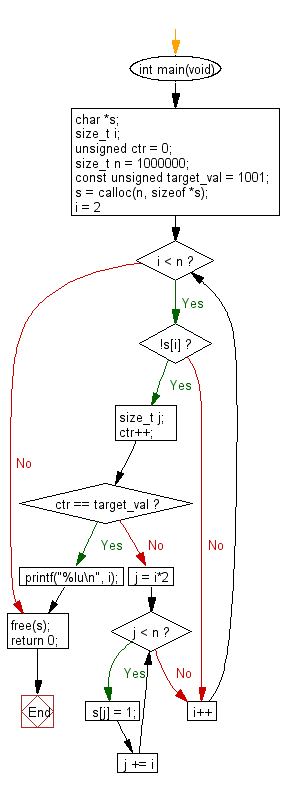﻿ C Program: Get the 1001st prime number - w3resource# C Exercises: Get the 1001st prime number

## C Programming Practice: Exercise-23 with Solution

By listing the first six prime numbers: 2, 3, 5, 7, 11, and 13, we can see that the 6th prime is 13.
The first six prime numbers are 2, 3, 5, 7, 11, and 13.
Write a C programming to get the 1001st prime number?

C Code:

``````#include <stdio.h>
int main(void)
{
char *s;
size_t i;
unsigned ctr = 0;
size_t n = 1000000;
const unsigned target_val = 1001;
s = calloc(n, sizeof *s);
for (i = 2; i < n; i++) {
if (!s[i]) {
size_t j;
ctr++;
if (ctr == target_val) {
printf("%lu\n", i);
break;
}
for (j = i*2; j < n; j += i) {
s[j] = 1;
}
}
}
free(s);
return 0;
}
``````

Sample Output:

```7927
```

Flowchart:## C Programming Code Editor:

What is the difficulty level of this exercise?

Test your Programming skills with w3resource's quiz.

﻿

## C Programming: Tips of the Day

Maximum value of int:

In C:

```#include <limits.h>
then use
int imin = INT_MIN; // minimum value
int imax = INT_MAX;```

or

```#include <float.h>

float fmin = FLT_MIN;  // minimum positive value
double dmin = DBL_MIN; // minimum positive value

float fmax = FLT_MAX;
double dmax = DBL_MAX;```

Ref : https://bit.ly/3fi8yk9